8 years, 14 days

## Checking linearly dependent of a polyno...

I want to test linearly dependence of a polynomial f on a list of polynomials F by additional condition on parametric coefficients of linear parametric polynomial (linear for variables not parameters). Please note that:

1. The polynomialand the members of are always homogenous in the variables.
2. The coefficients of f, the coefficients of the members of F are all always polynomials in the parameters or contant and the members of N and W are all always polynomials in the parameters.

For example let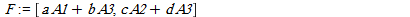and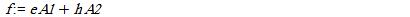(a,b,c,d,e,h are parameters and A1,A2,A3 are variables).

If I use PolyLinearCombo(F,f,{A1,A2,A3}) (see http://www.mapleprimes.com/questions/204469-How-Can-I-Find-The-Coefficients-Of-Linear#comment217621)then its output is false,[].

Now we let to condition sets for parameters as the following:

N:=[ebc+ahd]

W:=[a,c]

The elements of N must be zero means that ebc+ahd=0

and the elements of W are non-zero that is a<>0 and c <>0.

Let a=b=c=d=h=1 and e=-1. This specialization satisfy in the above condition sets N and W. By this specialization we have: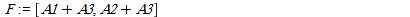and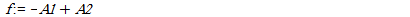Now if I use PolyLinearCombo(F,f,{A1,A2,A3}) then its output is true,[-1,1].

By this additional two condition sets I have to check that whether f is linearly independent of F or not. How can I do this without specialization? In fact I want an algorithm that its input is (null condition N, not-null condition W, list of polynomials F, a polynomial f, the set of variables) and its output is true and coefficients if f is linearly dependent of F w.r.t. null and not-null conditions N and W, else its output is false.

If the name of new procedure is ExtPolyLinearCombo andN:=[ebc+ahd]

W:=[a,c]

I want the output of

ExtPolyLinearCombo(N,W,F,f,{A1,A2,A3}) be true,[coefficients]

Thank you very much in advance.

## convert a multivariate polynomial into a...

At the first note that in this question all polynomials have parametric coefficients. Let F be a list of polynomials and f be a polynomial. I want to convert F and f into a linear homogeneous FF and ff resp. At the first I want to sortvthe monomials appears in F and f  w.r.t. a monomial order T and then replace by the new variables A_i.

For example if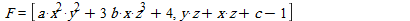and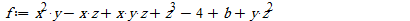(a,b,c are parameters and x,y,z are variables) then I want to convert F and f into FF and ff resp: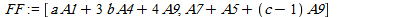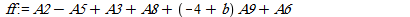please note that the variables appears in F and f are: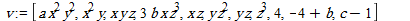where sorted by T=plex(x,y,z). Please note that we consider all constants and alone parameters (4, b-4, c-1) as A9. I want to convert v into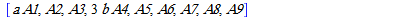and then F into FF and f into ff.

## Extract the coefficients of all monomial...

How can I extract the coefficients of all monomials in a multivariate polynomial?

For example if f=ax^2+bxy^3+2 then I want

coeff(f,x^2)=a

coeff(f,xy^3)=b

coeff(f,1/2)=4

coeff(f,1/10)=20 and...

## How can I find the coefficients of linea...

Maple

I know the Wronskian command. I want to use this command for detecting linearly dependence or independence of some polynomials. I know that the polynomials  are  linearly independent if the Wronskian is not zero.  Conversely, if the Wronskian vanishes  then the polynomials are linearly dependent. Now I want to know that how can I find the coefficient vector if the polynomials are dependent by Wronskian command?

For example if [f1,f2,f3] be a list of polynomials s.t. a1f1+a2f2+a3f3=0. How can I find a1, a2, a3?

## a polynomial is linear combination of so...

How  we can decide whether a polynomial f is a linear combination of some polynomials g1,...,gm?

For example if f=x^2+y^2 and g1=y+x^2 , g2=y^2-y then f=g1+g2.

﻿